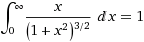Feynman is not too careful about issues of dx in his informal presentation. We have another source of information that restricts QED—it must explain why the wave theory of light gives the right numbers in so many situations. In fact it must explain light as well as Maxwell’s equations do. This should even give a theory of polarized light as well.

Suppose we have a plane barrier one unit away from light source A. We put a photon detector just behind a hole in the barrier. All would agree that the probability of detecting a photon is proportional to the solid angle subtended by the hole from the A. The distance of the hole from the point in the plane nearest A is r. The angle of the ray from the perpendicular is θ = tan−1 r. f = cos θ is the factor by which the apparent diameter of the hole is foreshortened viewed from A. f = 1/√(1+r2). The other factor in the probability of a photon reaching the detector is the reciprocal of the square of the distance, or 1/(1 + r2). In all the solid angle of the hole viewed from A is (1+r2)−3/2 da where da is the real area of the hole. The solid angle TA of the whole barrier should be 2π. We integrate over the entire plane thus:
TA = 0 0r(1+r2)−3/2dr dθ = 2π.
 Wolfram assures us that. (Actually ∫ x(1+x2)−3/2 dx = −(1+x2)−1/2 from which the definite integral easily follows.)
All that we have shown is that all the light that leaves the source towards the barrier, reaches the barrier.

We now move the detector from the hole to point B which is opposite A on the other side of the barrier. We have the same attenuation there and the total attenuation is also (1+r2)−3. The distance traveled on the two legs is 2(1+r2)½.

I think that I have now done the math to show that gradually removing the barrier gets you back to the situation if it had not been there. I still lack a coherent explanation, however. Actually I think I have only shown the following: If I erect a flat surface one unit away from a light source which absorbs all the light that strike it and reradiates it on the other side in a Lambertian pattern then the light intensity at the point of reflection of the source, is the same as without the surface. This is neither trivial nor profound.

To speak classically lets consider the partial differential wave equation:
2ψ/∂t2 = −∇ψ
The boundary condition at the barrier is absorbing and thus
∂ψ/∂z = ∂ψ/∂t when z=0 at the barrier—we have a black barrier.

To corroborate this boundary condition we try a known solution to ∂2ψ/∂t2 = −∇ψ:
ψ = cos (t + (x, y, z)∙v)
where p∙q is Gibb’s dot product and v is some constant unit vector. We need a better boundary condition.

Boundary Conditions